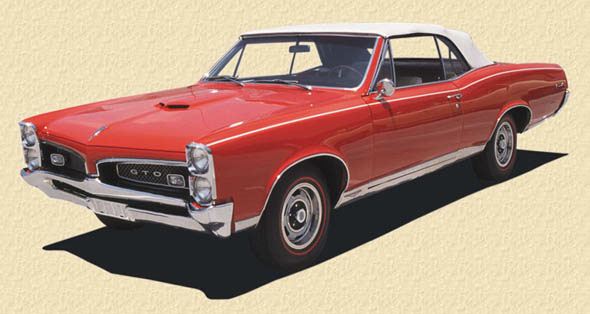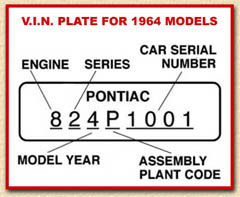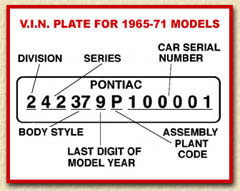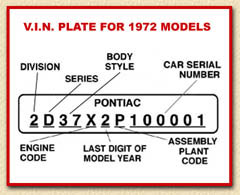## GTO VIN DecodingIn 1964, the serial number identification plate was attached to the left front body hinge pillar directly below the upper door hinge.1st digit – Engine: 6 = L6 8 = V8 (326 or 389)  2nd digit – Series: 0 = Tempest 1 = Tempest Custom 2 = Tempest LeMans 3rd digit – Model Year: 4 = 1964 4th digit – Assembly plant – B = Baltimore, MD D = Doraville, GA E = Linden, NJ F = Fremont, CA G = Framingham, MA M = Kansas City, MO P = Pontiac, MI R = Arlington, TX S = Southgate, CA U = Lordstown, OH X = Kansas City, KS  Last 6 digits – Production sequence starting at 1001 at all plants.

From 1965 to 1967 the serial number identification plate was attached to the left front body hinge pillar directly below the upper door hinge. From 1968 to 1971 it was attached to the top of the instrument panel on the driver’s side and is visible through the windshield from outside the car.1st digit – Division – 2 = Pontiac  2nd & 3rd digits – Series: 33 = Tempest (1965-70) 33 = LeMans T-37 (1971) 35 = Tempest Custom (1965-70) 33 = LeMans (1971) 37 = Tempest LeMans (1965-70) 37 = LeMans Sport (1971) 39 = Tempest Safari (1967-69) 42 = GTO (1966-71)
4th & 5th digits Body Code:
07 = 2-door sedan (post)
17 = 2-door coupe
27 = 2-door sedan (post)
35 = 4-door wagon, 2 seat
36 = 4-door wagon, 2 seat,
dual action tailgate
37 = 2-door coupe
39 = 4-door coupe
46 = 4-door wagon, 3 seat,
dual action tailgate
67 = 2-door convertible
69 = 4-door sedan

6th digit – Model Year:
5 = 1965
6 = 1966
7 = 1967
8 = 1968
9 = 1969
0 = 1970
1 = 1971

7th digit – Assembly plant:
A = Atlanta, GA
B = Baltimore, MD
C = Southgate, CA
D = Doraville, GA
E = Linden, NJ
G = Framingham, MA
K = Kansas City, MO
P = Pontiac, MI
R= Arlington, TX
U = Lordstown, OH
V = Bloomfield, IL
X = Kansas City, KS
Z = Fremont, CA

Last 6 digits Production sequence starting at 600001
at all plants, except V8 models which started at 100001.

In 1972, the serial number identification plate was attached to the top of the instrument panel on the driver’s side and is visible through the windshield from outside the car.1st digit – Division – 2 = Pontiac  2nd digit – Series: D = LeMans G = Luxury LeMans
3rd and 4th digits – Body Style:
27 = 2-door sedan (post)
36 = 4-door wagon, 2 seat
37 = 2-door coupe
39 = 4-door coupe
46 = 4-door wagon, 3 seat
67 = 2-door convertible
69 = 4-door sedan

5th digit – Engine Code:
D = 250 1-bbl L6 110 hp
M = 350 2-bbl V8 160 hp
N = 350 2-bbl V8 160 hp (dual exh.)
R = 400 2-bbl V8 180 hp
S = 400 4-bbl V8 200 hp (single exh.)
T = 400 4-bbl V8 250 hp (dual exh.)
Y = 455 4-bbl V8 250 hp (dual exh.)
X = 455 4-bbl V8 300 hp (455 HO)

6th digit – Model Year:
2 = 1972

7th digit – Assembly plant –
A = Atlanta, GA
C = Southgate, CA
D = Doraville, GA
G = Framingham, MA
L = Van Nuys, CA
N = Norwood, OH
P = Pontiac, MI
R = Arlington , TX
W = Willow Run, MI
X = Kansas City, KS
Z = Fremont, CA
2 = St. Therese, Quebec, Canada

Last 6 digits – Production sequence starting at 100001 at all plants.

## Subscribe to our mailing list

* indicates required